Anúncio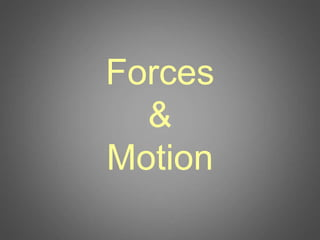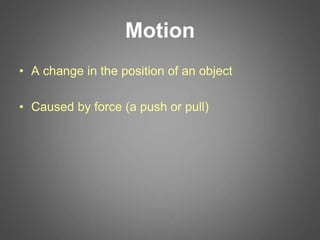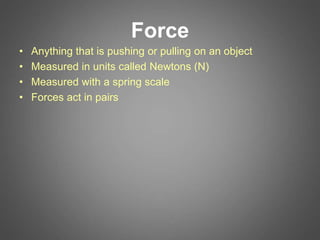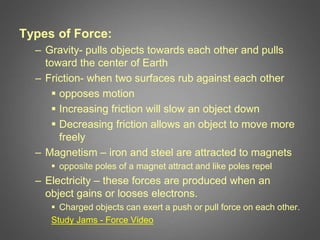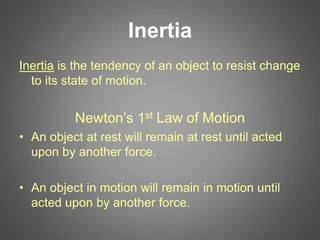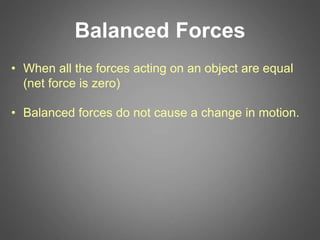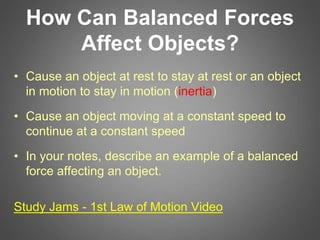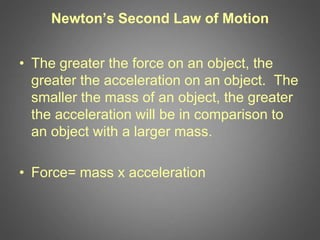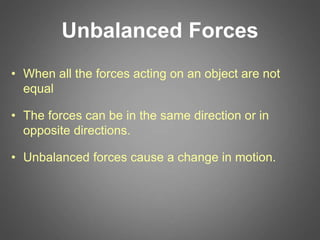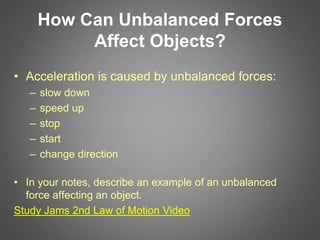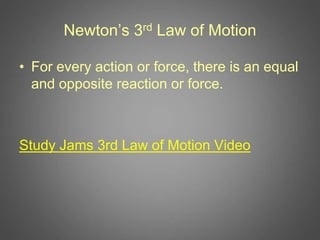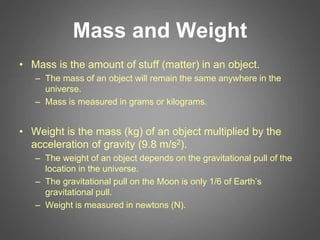1 de 12
Anúncio

### 1 Force and Motion Lesson.ppt

1. Forces & Motion
2. Motion • A change in the position of an object • Caused by force (a push or pull)
3. Force • Anything that is pushing or pulling on an object • Measured in units called Newtons (N) • Measured with a spring scale • Forces act in pairs
4. Types of Force: – Gravity- pulls objects towards each other and pulls toward the center of Earth – Friction- when two surfaces rub against each other  opposes motion  Increasing friction will slow an object down  Decreasing friction allows an object to move more freely – Magnetism – iron and steel are attracted to magnets  opposite poles of a magnet attract and like poles repel – Electricity – these forces are produced when an object gains or looses electrons.  Charged objects can exert a push or pull force on each other. Study Jams - Force Video
5. Inertia Inertia is the tendency of an object to resist change to its state of motion. Newton’s 1st Law of Motion • An object at rest will remain at rest until acted upon by another force. • An object in motion will remain in motion until acted upon by another force.
6. Balanced Forces • When all the forces acting on an object are equal (net force is zero) • Balanced forces do not cause a change in motion.
7. How Can Balanced Forces Affect Objects? • Cause an object at rest to stay at rest or an object in motion to stay in motion (inertia) • Cause an object moving at a constant speed to continue at a constant speed • In your notes, describe an example of a balanced force affecting an object. Study Jams - 1st Law of Motion Video
8. Newton’s Second Law of Motion • The greater the force on an object, the greater the acceleration on an object. The smaller the mass of an object, the greater the acceleration will be in comparison to an object with a larger mass. • Force= mass x acceleration
9. Unbalanced Forces • When all the forces acting on an object are not equal • The forces can be in the same direction or in opposite directions. • Unbalanced forces cause a change in motion.
10. How Can Unbalanced Forces Affect Objects? • Acceleration is caused by unbalanced forces: – slow down – speed up – stop – start – change direction • In your notes, describe an example of an unbalanced force affecting an object. Study Jams 2nd Law of Motion Video
11. Newton’s 3rd Law of Motion • For every action or force, there is an equal and opposite reaction or force. Study Jams 3rd Law of Motion Video
12. Mass and Weight • Mass is the amount of stuff (matter) in an object. – The mass of an object will remain the same anywhere in the universe. – Mass is measured in grams or kilograms. • Weight is the mass (kg) of an object multiplied by the acceleration of gravity (9.8 m/s2). – The weight of an object depends on the gravitational pull of the location in the universe. – The gravitational pull on the Moon is only 1/6 of Earth’s gravitational pull. – Weight is measured in newtons (N).
Anúncio